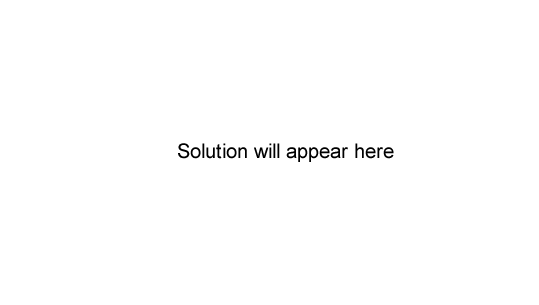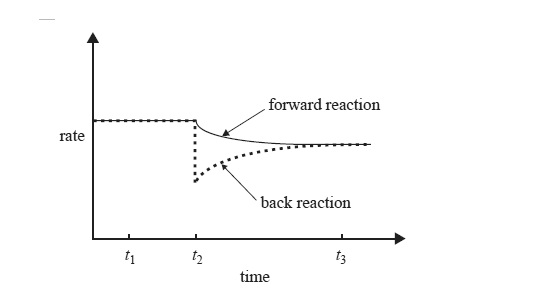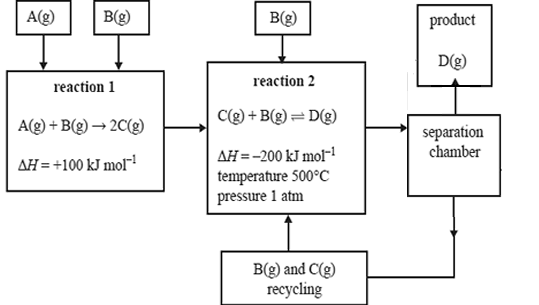Equilibrium chemistry 2009 VCE

The concentrations of reactants and products were studied for the following reaction.
H2(g) + F2(g) <=> 2HF(g) ; K = 313 at 25°C
In an experiment, the initial concentrations of the gases were
[H2] = 0.0200 M, [F2] = 0.0100 M and [HF] = 0.400 M
When the reaction reaches equilibrium at 25°C, the concentration of HF will be
A. 0.400 M
B. 0.420 M
C. between 0.400 M and 0.420 M
D. less than 0.400 M

SolutionThe anaesthetic, nitrous oxide,N2O, decomposes to form an equilibrium mixture of N2O, N2 and O2 according to the following equation.
2N2O(g) <=>2N2(g) + O2(g)
At 25°C, K = 7.3 × 1037 M and at 40°C, K = 2.7 × 1036 M
What valid conclusion can be made from this?
A. The equilibrium concentrations of N2 and O2 are equal at 25°C.
B. The equilibrium concentration of N2O is higher at 25°C than at 40°C.
C. N2O is less stable at the higher temperature.
D. The forward reaction is exothermic.

SolutionWrite an equation for the equilibrium reaction of ammonium ions with water.

SolutionWhile keeping the temperature constant, the chemist makes a change to a solution of ammonium ions in water that is initially at equilibrium. The graph on the right shows the effect of this change, which was made at time t2, on the rates of the forward and back reactions.

What could have caused the change that occurred at time t2? Explain why the rate of the back reaction is affected by this change.

Solution

Would the value of the equilibrium constant at time t3 be less than, equal to or greater than the value of the equilibrium constant at time t1? Select the correct response.

SolutionDimethyl ether, CH3OCH3, is used as an environmentally friendly propellant in spray cans. It can be synthesised from methanol according to the following equation.
2CH3OH(g) <=>CH3OCH3(g) + H2O(g); /\H = –24 kJ mol–1
The equilibrium constant, K, for this reaction at 350°C is 5.74.
a. Write an expression for K for this reaction.

Solution

b. Calculate the value of K at 350°C for the following reaction.
CH3OCH3(g) + H2O(g) <=> 2CH3OH(g)

Solution

c. Methanol is pumped into an empty 20.0 L reactor vessel. At equilibrium the vessel contains 0.340 mol of methanol at 350°C.
i. Calculate the concentration, in mol L−1, of methanol at equilibrium.
Solution
ii. Calculate the amount, in mol, of dimethyl ether present at equilibrium.
Solution
iii. Calculate the amount, in mol, of methanol initially pumped into the reaction vessel.
SolutionA particular industrial process involves the steps shown on the right. It is possible to alter the temperature and pressure at which reaction 2 occurs.
In the table below, indicate what effect the following changes to temperature and pressure would have on the rate, equilibrium yield and value of the equilibrium constant, K, for reaction 2.

 Would the rate of reaction 2 become higher, lower or remain unchanged? Would the equilibrium yield of reaction 2 become higher, lower or remain unchanged? Would the value of the equilibrium constant, K, of reaction 2 become higher, lower or remain unchanged? The temperature of reaction 2 is lowered to 150°C. higher lower unchanged higher lower unchanged higher lower unchanged The pressure of reaction 2 is increased to 5 atm by pumping more B(g) and C(g) into the reaction vessel, at constant temperature. higher lower unchanged higher lower unchanged higher lower unchanged

Solution